Courses

# Test: Subtraction - 2

## 20 Questions MCQ Test Mathematics (Maths) for Class 1 | Test: Subtraction - 2

Description
This mock test of Test: Subtraction - 2 for Class 1 helps you for every Class 1 entrance exam. This contains 20 Multiple Choice Questions for Class 1 Test: Subtraction - 2 (mcq) to study with solutions a complete question bank. The solved questions answers in this Test: Subtraction - 2 quiz give you a good mix of easy questions and tough questions. Class 1 students definitely take this Test: Subtraction - 2 exercise for a better result in the exam. You can find other Test: Subtraction - 2 extra questions, long questions & short questions for Class 1 on EduRev as well by searching above.
QUESTION: 1

Solution:
QUESTION: 2

Solution:
QUESTION: 3

### What is 10-5?

Solution:
QUESTION: 4

Find the missing number :

7 - 1 = ___

Solution:
QUESTION: 5

Subtract

9 from 9

Solution:
QUESTION: 6

Ravi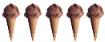ice-creams. He gives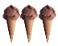to his friend.

How many ice-creams are left with Ravi?

Solution:
QUESTION: 7

8 dolls - 3 dolls = _____

Solution:
QUESTION: 8

Subtract :

5 - 3

Solution:
QUESTION: 9

Priya has 99 sweets. She gave 46 to her sister. How many sweets will be left with Priya?

Solution:
QUESTION: 10

Subtract :

8 - 6

Solution:
QUESTION: 11

6 - 2 = ?

Solution:
QUESTION: 12

Which of the following is the same as 13-3?

Solution:
QUESTION: 13

Find the missing number :

8 - ___ = 4

Solution:
QUESTION: 14

Find the missing number :

8 - ___ = 3

Solution:
QUESTION: 15

45 oranges are in a basket. 34 were put into a bag. Find the number of oranges remaining in the basket.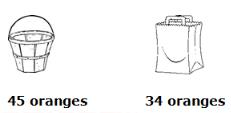Solution:
QUESTION: 16

Subtract 4 from 7

Solution:
QUESTION: 17

Subtract :

3 - 2

Solution:
QUESTION: 18

Subtract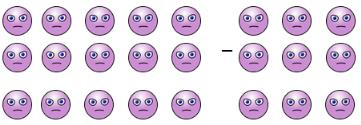Solution:
QUESTION: 19

Subtract :

9 - 2

Solution:
QUESTION: 20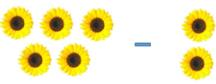Solution: